# The GLM Procedure

### Specification of ESTIMATE Expressions

Subsections:

Consider the model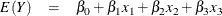The corresponding MODEL statement for PROC GLM is

model y=x1 x2 x3;


To estimate the difference between the parameters for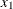and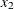,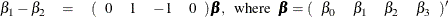you can use the following ESTIMATE statement:

estimate 'B1-B2'  x1 1  x2 -1;


To predict y at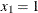,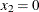, and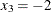, you can estimate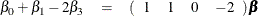with the following ESTIMATE statement:

estimate 'B0+B1-2B3' intercept 1 x1 1 x3 -2;


Now consider models involving classification variables such as

model y=A B A*B;


with the associated parameters: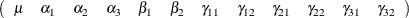The LS-mean for the first level of A is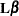, where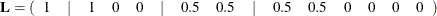You can estimate this with the following ESTIMATE statement:

estimate 'LS-mean(A1)' intercept 1 A 1 B 0.5 0.5 A*B 0.5 0.5;


Note in this statement that only one element ofis specified following the A effect, even though A has three levels. Whenever the list of constants following an effect name is shorter than the effect’s number of levels, zeros are used as the remaining constants. (If the list of constants is longer than the number of levels for the effect, the extra constants are ignored, and a warning message is displayed.)

To estimate the A linear effect in the preceding model, assuming equally spaced levels for A, you can use the following: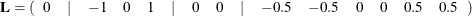The ESTIMATE statement for thisis written as

     estimate 'A Linear' A -1 0 1;


If you do not specify the elements offor an effect that contains a specified effect, then the elements of the specified effect are equally distributed over the corresponding levels of the higher-order effect. In addition, if you specify the intercept in an ESTIMATE or CONTRAST statement, it is distributed over all classification effects that are not contained by any other specified effect.

The distribution of lower-order coefficients to higher-order effect coefficients follows the same general rules as in the LSMEANS statement, and it is similar to that used to construct Type IV tests. In the previous example, the –1 associated with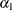is divided by the number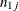of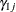parameters; then eachcoefficient is set to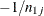. The 1 associated with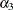is distributed among the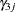parameters in a similar fashion. In the event that an unspecified effect contains several specified effects, only that specified effect with the most factors in common with the unspecified effect is used for distribution of coefficients to the higher-order effect.

Numerous syntactical expressions for the ESTIMATE statement were considered, including many that involved specifying the effect and level information associated with each coefficient. For models involving higher-level effects, the requirement of specifying level information can lead to very bulky specifications. Consequently, the simpler form of the ESTIMATE statement described earlier was implemented.

The syntax of this ESTIMATE statement puts a burden on you to know a priori the order of the parameter list associated with each effect. You can use the ORDER= option in the PROC GLM statement to ensure that the levels of the classification effects are sorted appropriately.

Note: If you use the ESTIMATE statement with unspecified effects, use the E option to make sure that the actualconstructed by the preceding rules is the one you intended.

#### A Check for Estimability

Eachis checked for estimability using the relationship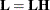, where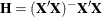. Thevector is declared nonestimable, if for any i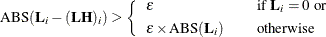where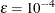by default; you can change this with the SINGULAR= option. Continued fractions (like 1/3) should be specified to at least six decimal places, or the DIVISOR parameter should be used.